# INS 1. For the following reactions, write the balanced molecular equation, complete ionic equation, and net...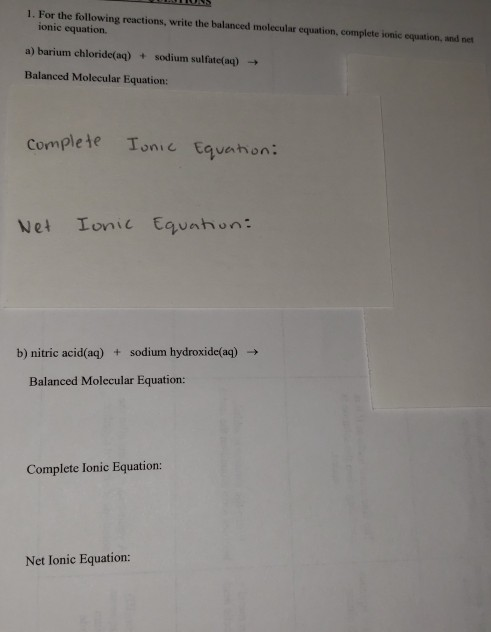INS 1. For the following reactions, write the balanced molecular equation, complete ionic equation, and net ionic equation a) barium chloride(aq) + sodium sulfate(aq) + Balanced Molecular Equation: Complete Ionic Equation: Net Ionic Equation: b) nitric acid(aq) + sodium hydroxide(aq) → Balanced Molecular Equation: Complete Ionic Equation: Net Ionic Equation: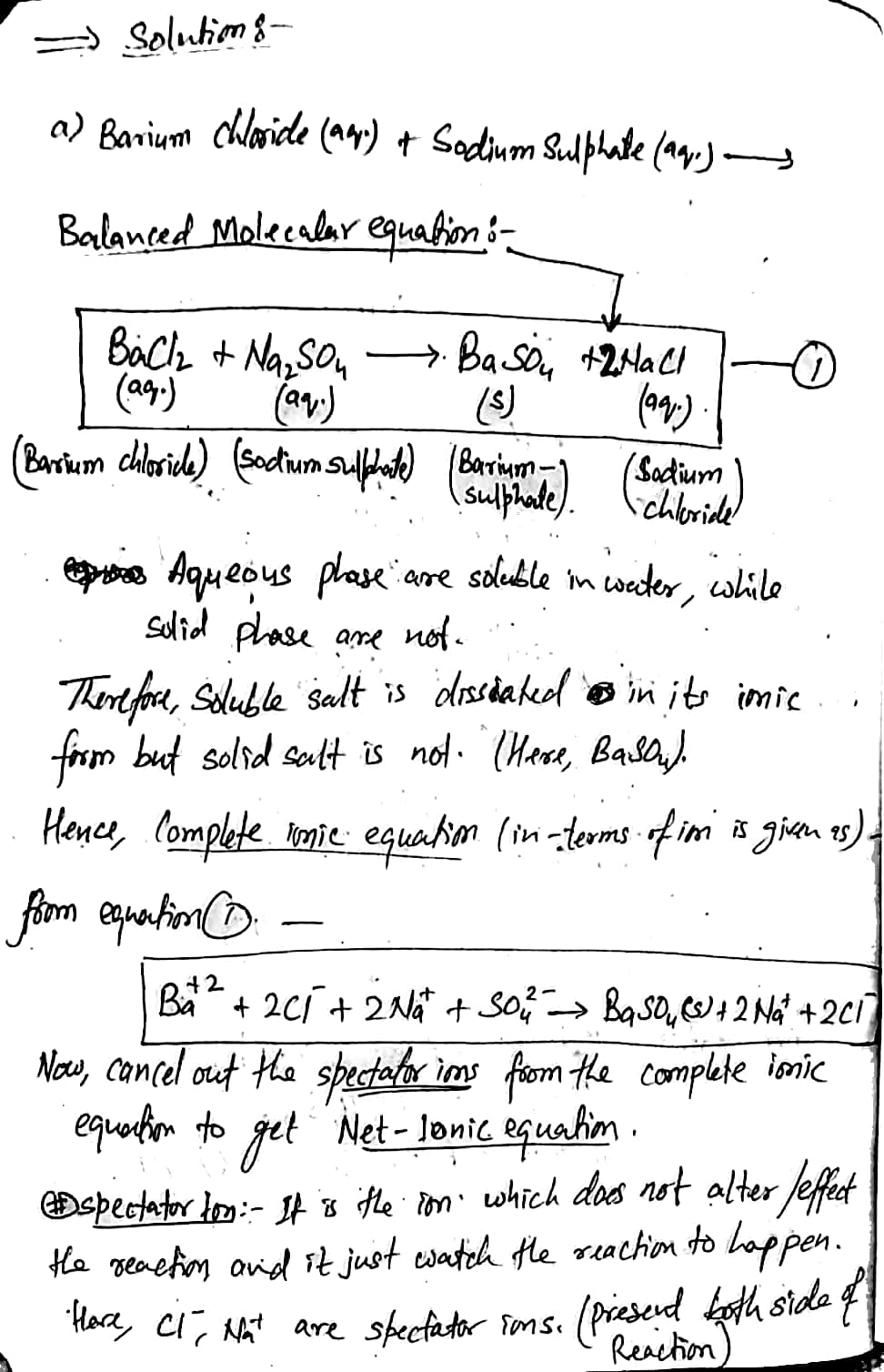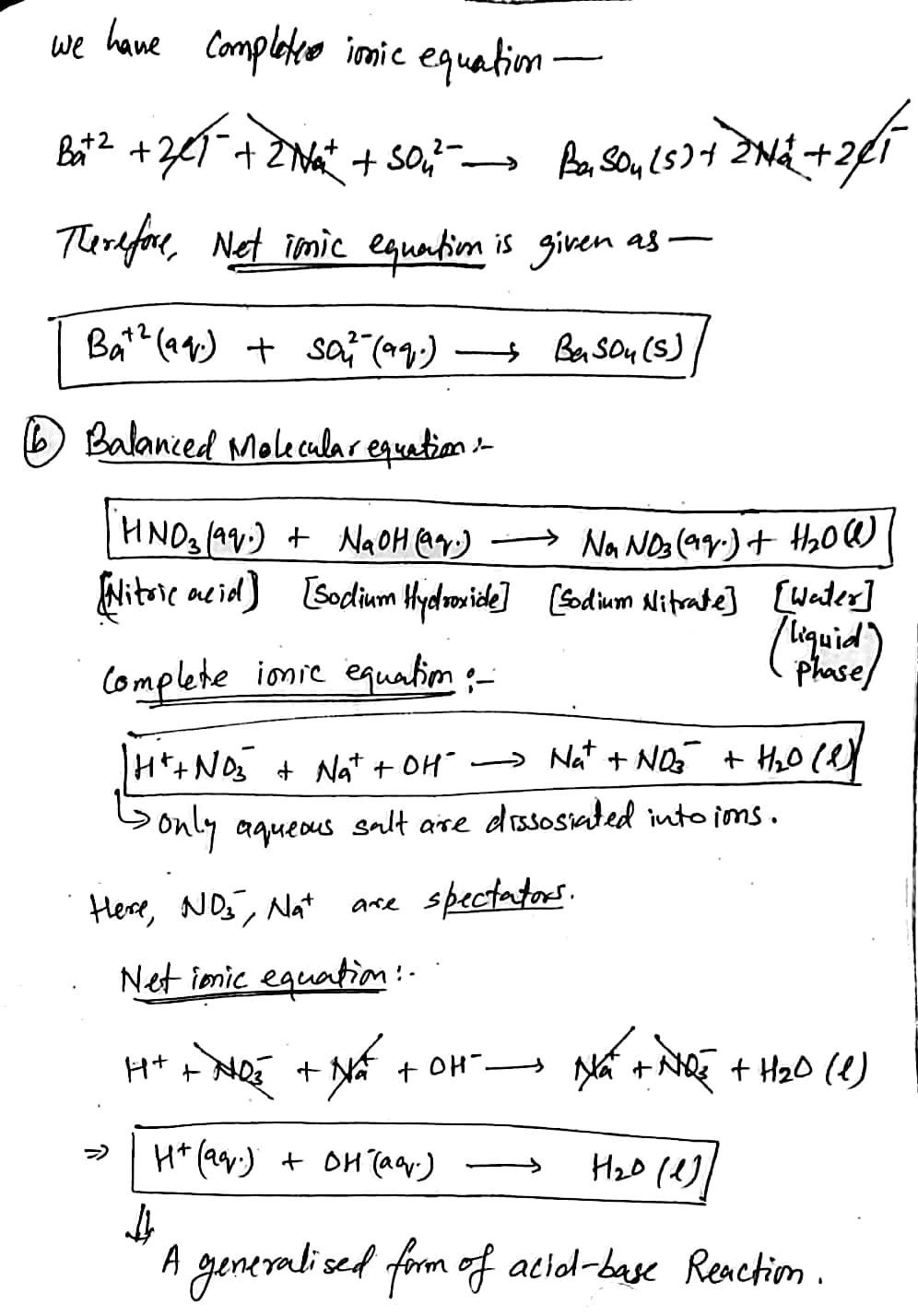thanks, please upvote it

#### Earn Coin

Coins can be redeemed for fabulous gifts.

Similar Homework Help Questions
• ### 1) write molecular, complete ionic, and net ionic equations for the reactions that occur, if any, when solutions of the...

1) write molecular, complete ionic, and net ionic equations for the reactions that occur, if any, when solutions of the following substances are mixed: a) nitric acid and barium carbonate, b) zinc chloride and lead nitrate, c) acetic acid and sodium hydroxide, d) calcium nitrate and sodium carbonate, e) ammonium chloride and potassium hydroxide.

• ### Writing Balanced Molecular, Ionic, And Net Ionic Equations Write Balanced Net-Ionic Equations for the following Reactions...

Writing Balanced Molecular, Ionic, And Net Ionic Equations Write Balanced Net-Ionic Equations for the following Reactions in Aqueous Solution: 1.      Copper metal (Cu(s)) is immersed in an aqueous solution of silver nitrate (AgNO3). The solution turns light blue and a silver coating appears on the copper. 2.      Dilute solutions of antimony(III) chloride and sodium sulfide are mixed to give a precipitate. 3.      Dilute solutions of silver nitrate and potassium iodide are combined and give a yellow precipitate. 4.      Dilute solutions...

• ### Please complete the following reactions, and show the balanced molecular, complete ionic, and net ionic equations:...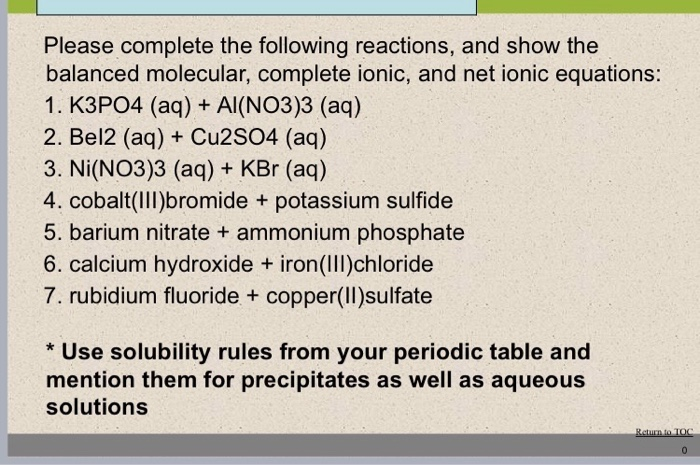Please complete the following reactions, and show the balanced molecular, complete ionic, and net ionic equations: 1. K3PO4 (aq) + Al(NO3)3 (aq) 2. Bel2 (aq) Cu2SO4 (aq) 3. Ni(NO3)3 (aq) + KBr (aq) 4. cobalt(lll)bromide potassium sulfide 5. barium nitrate ammonium phosphate 6. calcium hydroxide iron(Il)chloride 7. rubidium fluoride + copper(II)sulfate Use solubility rules from your periodic table and mention them for precipitates as well as aqueous solutions Return to TOC

• ### whats the answers 1. Write molecular, complete ionic, and net jonic equations for the reactions that...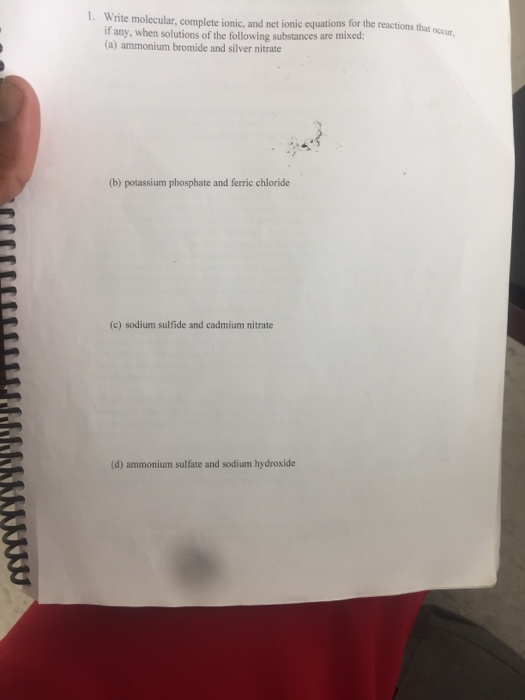whats the answers 1. Write molecular, complete ionic, and net jonic equations for the reactions that ove if any, when solutions of the following substances are mixed: (a) ammonium bromide and silver nitrate (b) potassium phosphate and ferric chloride (c) sodium sulfide and cadmium nitrate (d) ammonium sulfate and sodium hydroxide QUESTIONS: 1. Write molecular, complete ionic, and net ionic equations for the reactions that occur, if any, when solutions of the following substances are mixed: (a) ammonium bromide and...

• ### Write the balanced molecular equation, full ionic equation, and net ionic equation for the following reactions:...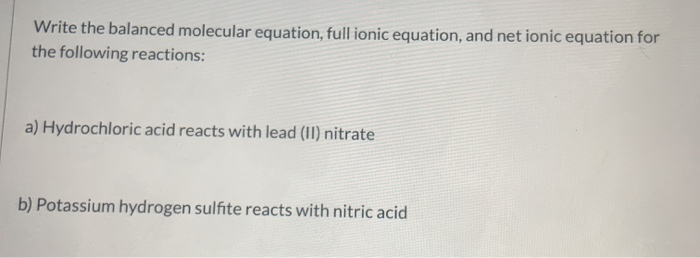Write the balanced molecular equation, full ionic equation, and net ionic equation for the following reactions: a) Hydrochloric acid reacts with lead (II) nitrate b) Potassium hydrogen sulfite reacts with nitric acid

• ### Write the balanced molecular equation, full ionic equation, and net ionic equation for the following reactions:...

Write the balanced molecular equation, full ionic equation, and net ionic equation for the following reactions: a) Hydrochloric acid reacts with lead (II) nitrate b) Potassium hydrogen sulfite reacts with nitric acid

• ### BWrite the molecular equation, total ionic equation, and net ionic equation for the following precipitation reaction. B...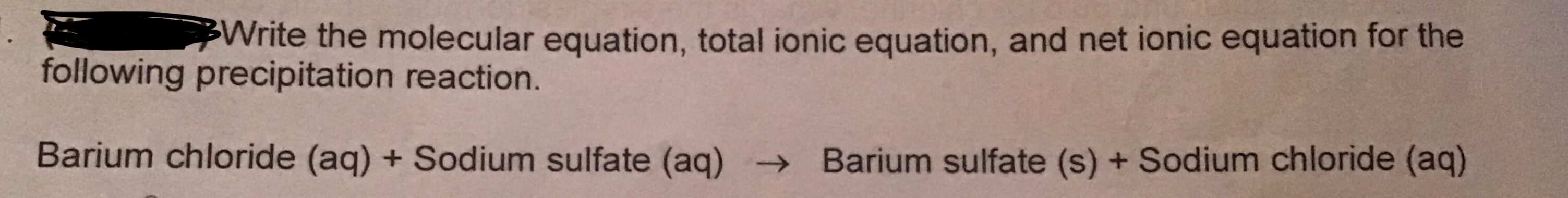BWrite the molecular equation, total ionic equation, and net ionic equation for the following precipitation reaction. Barium chloride (aq) + Sodium sulfate (aq) + Barium sulfate (s) + Sodium chloride (aq)

• ### 18. For each of the following, write a balanced equation and a net ionic equation, if...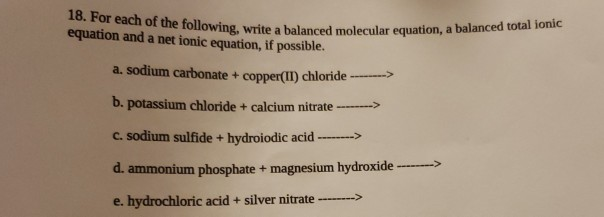18. For each of the following, write a balanced equation and a net ionic equation, if possible. howing, write a balanced molecular equation, a balanced total ionic a. sodium carbonate + copper(II) chloride -----> b. potassium chloride + calcium nitrate ------> C. sodium sulfide + hydroiodic acid -------> d. ammonium phosphate + magnesium hydroxide -----> e. hydrochloric acid + silver nitrate ------->

• ### What's the complete molecular equation, net ionic equation, and spectator ion(s) of each of the following...

What's the complete molecular equation, net ionic equation, and spectator ion(s) of each of the following reactions. 1.) hydrochloric acid and ammonium carbonate 2.) hydrochloric acid and magnesium metal 3.) hydrochloric acid and lead(ii) nitrate 4.) hydrochloric acid and sodium hydroxide 5.) hydrochloric acid and silver nitrate 6.) hydrochloric acid and ammonium sulfate 7.) barium nitrate and ammonium sulfate 8.) sodium hydroxide and copper(ii) nitrate 9.) aluminum nitrate and sodium hydroxide 10.) hydrochloric acid and copper metal

• ### A) Write balanced (a) molecular, (b) total ionic, and (c) net ionic equations for the following...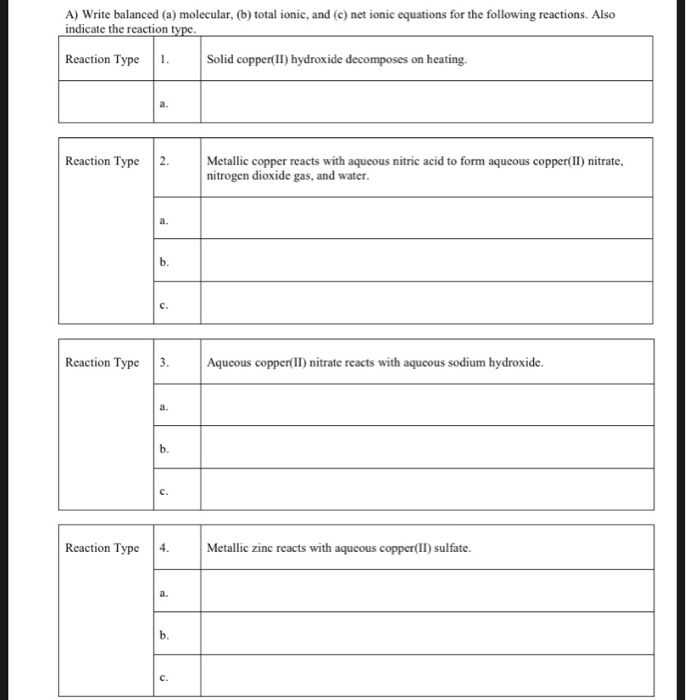A) Write balanced (a) molecular, (b) total ionic, and (c) net ionic equations for the following reactions. Also indicate the reaction type. Reaction Type 1. Solid copper(II) hydroxide decomposes on heating. Reaction Type 2. Metallic copper reacts with aqueous nitric acid to form aqueous copper(II) nitrate, nitrogen dioxide gas, and water. Reaction Type 3. Aqueous copper(II) nitrate reacts with aqueous sodium hydroxide. Reaction Type 4. Metallic zinc reacts with aqueous copper(II) sulfate.